Math - 2018 Past Year Paper

# Math - 2018 Past Year Paper

Test Description

## 60 Questions MCQ Test IIT JAM Past Year Papers and Model Test Paper (All Branches) | Math - 2018 Past Year Paper

Math - 2018 Past Year Paper for IIT JAM 2022 is part of IIT JAM Past Year Papers and Model Test Paper (All Branches) preparation. The Math - 2018 Past Year Paper questions and answers have been prepared according to the IIT JAM exam syllabus.The Math - 2018 Past Year Paper MCQs are made for IIT JAM 2022 Exam. Find important definitions, questions, notes, meanings, examples, exercises, MCQs and online tests for Math - 2018 Past Year Paper below.
Solutions of Math - 2018 Past Year Paper questions in English are available as part of our IIT JAM Past Year Papers and Model Test Paper (All Branches) for IIT JAM & Math - 2018 Past Year Paper solutions in Hindi for IIT JAM Past Year Papers and Model Test Paper (All Branches) course. Download more important topics, notes, lectures and mock test series for IIT JAM Exam by signing up for free. Attempt Math - 2018 Past Year Paper | 60 questions in 180 minutes | Mock test for IIT JAM preparation | Free important questions MCQ to study IIT JAM Past Year Papers and Model Test Paper (All Branches) for IIT JAM Exam | Download free PDF with solutions
 1 Crore+ students have signed up on EduRev. Have you?
Math - 2018 Past Year Paper - Question 1

### Which one of the following is True?

Math - 2018 Past Year Paper - Question 2

### Let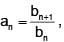where  b1 = 1, b2 = 1 and bn+2 = bn + bn+1,Then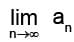is:

Math - 2018 Past Year Paper - Question 3

### If {v1, v2, v3} is a linearly independent set of vectors in a vector space over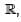then which one of the following sets is also linearly independent?

Math - 2018 Past Year Paper - Question 4

Let a be a positive real number. If f is a continuous and even function defined on the interval [–a, a], then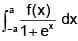is equal to :

Math - 2018 Past Year Paper - Question 5

The tangent plane to the surface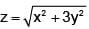at (1, 1, 2) is given by

Math - 2018 Past Year Paper - Question 6

In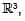, the cosine of the acute angle between the surfaces x2 + y2 + z2 - 9 = 0 and z - x2 - y2 + 3 = 0 at the point (2, 1, 2) is :

Math - 2018 Past Year Paper - Question 7

Let f :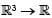be a scalar field,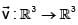be a vector filed and let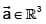be a constant vector. If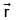represents the position vector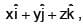then which one of the following is FALSE?

Math - 2018 Past Year Paper - Question 8

In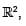, the family of trajectories orthogonal to the family of asteroids x2/3 + y2/3 = a2/3 is given by

Math - 2018 Past Year Paper - Question 9

Consider the vector space V over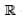of polynomial functions of degree less then or equal to 3 defined on. Let T : V → V be defined by (Tf)(x) = f(x) – xf’(x). Then the rank of T is

Math - 2018 Past Year Paper - Question 10

Let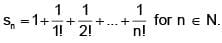Then which one of the following is True for the sequence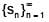Math - 2018 Past Year Paper - Question 11

Let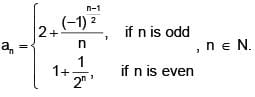Then which one of the following is TRUE?

Math - 2018 Past Year Paper - Question 12

Let a, b, c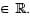. Which of the following values of a, b, c do NOT result in the convergence of the series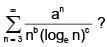Math - 2018 Past Year Paper - Question 13

Let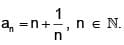Then the sum of the series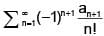is:

Math - 2018 Past Year Paper - Question 14

Let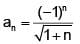and let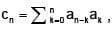where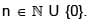Then which one of the following is true?

Math - 2018 Past Year Paper - Question 15

Suppose that f, g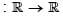are differentiable functions such that f is strictly increasing and g is strictly decreasing. Define p(x) = f(g(x)) and q(x) = g(f(x)),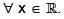Then, for t > 0, the sign of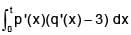is :

Math - 2018 Past Year Paper - Question 16

ForThen which one of the following is false?

Math - 2018 Past Year Paper - Question 17

Let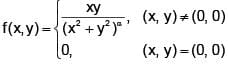Then which one of the following is true for f at the point (0, 0)?

Math - 2018 Past Year Paper - Question 18

Let a, b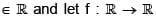be a thrice differentiable function. If z = eu f(v), where u = ax + by and v = ax – by, then which one of the following is true?

Math - 2018 Past Year Paper - Question 19

Consider the region D in the yz plane bounded by the line y = 1/2 and the curve y2 + z2 = 1, where y≥0. If the region D is revolved about the z- axis in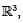, then the volume of the resulting solid is :

Math - 2018 Past Year Paper - Question 20

If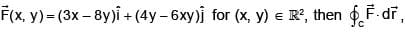where C is the boundary of the triangular region bounded by the lines x = 0, y = 0 and x + y = 1 oriented in the anti- clockwise direction, is :

Math - 2018 Past Year Paper - Question 21

Let U, V and W be finite dimensional real vector spaces, T : U → V, S : V → W and P : W → U be linear transformations. If range (ST) = nullspace (P), nullspace (ST) = range (P) and rank (T) = rank (S), then which one of the following is true?

Math - 2018 Past Year Paper - Question 22

Let y(x) be the solution of the differential equation dy/dx + = , for x ≥ 0, y(0) = 0, where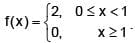Then y(x) =

Math - 2018 Past Year Paper - Question 23

An integrating factor of the differential equation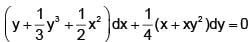is:

Math - 2018 Past Year Paper - Question 24

A particular integral of the differential equation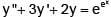is:

Math - 2018 Past Year Paper - Question 25

Let G be a group satisfying the property that f :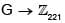is a homomorphism implies f(g) = 0,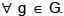Then a possible group G is :

Math - 2018 Past Year Paper - Question 26

Let H be the quotient group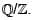Consider the following statements.
I. Every cyclic subgroup of H is finite.
II. Every finite cyclic group is isomorphic to a subgroup of H.
Which one of the following holds?

Math - 2018 Past Year Paper - Question 27

Let I denote the 4 × 4 identity matrix. If the roots of the characteristic polynomial of a 4 × 4 matrix M are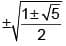, then M8 =

Math - 2018 Past Year Paper - Question 28

Consider the groupunder component- wise addition. Then which of the following is a subgroup of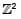?

Math - 2018 Past Year Paper - Question 29

Let f :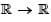be a function and let J be a bounded open interval in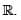Define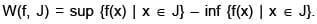Which one of the following is false?

Math - 2018 Past Year Paper - Question 30

For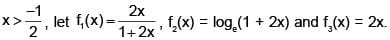Then which one of the following is true?

*Multiple options can be correct
Math - 2018 Past Year Paper - Question 31

Let f :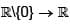be defined by f ( x) =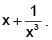On which of the following interval(s) is f one- one?

*Multiple options can be correct
Math - 2018 Past Year Paper - Question 32

The solution(s) of the differential equation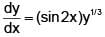satisfying y(0) = 0 is (are)

*Multiple options can be correct
Math - 2018 Past Year Paper - Question 33

Suppose f, g, h are permutations of the set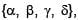where
f interchanges ∝ and β but fixes γ and δ
g interchanges β and γ but fixes ∝ and δ,
h interchanges γ and δ but fixes ∝ and β.
Which of the following permutations interchange(s) ∝ and δ but fix(es) β and γ?

*Multiple options can be correct
Math - 2018 Past Year Paper - Question 34

Let P and Q be two non- empty disjoint subsets of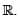Which of the following is (are) false?

*Multiple options can be correct
Math - 2018 Past Year Paper - Question 35

Let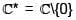denote the group of non- zero complex numbers under multiplication. Suppose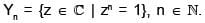Which of the following is (are) subgroup(s) of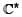?

*Multiple options can be correct
Math - 2018 Past Year Paper - Question 36

Suppose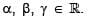Consider the following system of linear equations. x + y + z = α, x + βy + z = γ, x + y + αz = β. If this system has at least one solution, then which of the following statements is (are) true?

*Multiple options can be correct
Math - 2018 Past Year Paper - Question 37

Let m,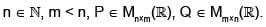Then which of the following is (are) Not possible?

*Multiple options can be correct
Math - 2018 Past Year Paper - Question 38

One among the following is the correct explanation of pedal equation of an polar curve, r = f (θ), p = r sin(∅) (where p is the length of the perpendicular from the pole to the tangent & ∅ is the angle made by tangent to the curve with vector drawn to curve from pole)is _______.

Detailed Solution for Math - 2018 Past Year Paper - Question 38

It is expressed in terms of p & r only

where p = r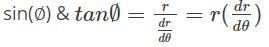& r = f (θ) or after solving we get direct relationship between p & r as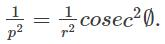*Multiple options can be correct
Math - 2018 Past Year Paper - Question 39

Which of the following subsets of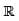is (are) connected?

*Multiple options can be correct
Math - 2018 Past Year Paper - Question 40

Let S be a subset of ¡ such that 2018 is an interior point of S. Which of the following is (are) true?

*Answer can only contain numeric values
Math - 2018 Past Year Paper - Question 41

The order of the element (1 2 3)(2 4 5)(4 5 6) in the group S6 is _______ .

*Answer can only contain numeric values
Math - 2018 Past Year Paper - Question 42

Let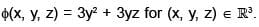Then the absolute value of the directional derivative of φ in the direction of the line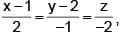at the point (1, – 2, 1) is _______ .

*Answer can only contain numeric values
Math - 2018 Past Year Paper - Question 43

Let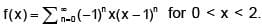Then the value of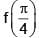is _______ .

*Answer can only contain numeric values
Math - 2018 Past Year Paper - Question 44

Let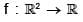be given by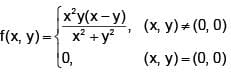Then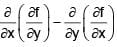at the point (0, 0) is _______ .

*Answer can only contain numeric values
Math - 2018 Past Year Paper - Question 45

Let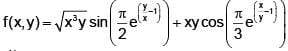for (x, y)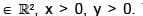Then fx(1, 1) + fy(1, 1) = _______ .

*Answer can only contain numeric values
Math - 2018 Past Year Paper - Question 46

Let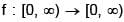be continuous on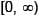and differentiable on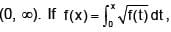then f(6) = _______ .

*Answer can only contain numeric values
Math - 2018 Past Year Paper - Question 47

Let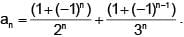Then the radius of convergence of the power series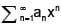about x = 0 is _______ .

*Answer can only contain numeric values
Math - 2018 Past Year Paper - Question 48

Let A6 be the group of even permutations of 6 distinct symbols. Then the number of elements of order 6 in A6 is _______ .

*Answer can only contain numeric values
Math - 2018 Past Year Paper - Question 49

Let W1 be the real vector space of all 5 × 2 matrices such that the some of the entries in each row is zero. Let W2 be the real vector space of all 5 × 2 matrices such that the sum of the entries in each column is zero. Then the dimension of the space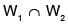is _______ .

*Answer can only contain numeric values
Math - 2018 Past Year Paper - Question 50

The coefficient of x4 in the power series expansion of esin x about x = 0 is _______ (correct up to three decimal places).

*Answer can only contain numeric values
Math - 2018 Past Year Paper - Question 51

Let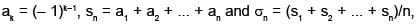where k,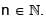Then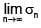is _______ . (correct up to one decimal places).

*Answer can only contain numeric values
Math - 2018 Past Year Paper - Question 52

Let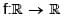be such that f" is continuous on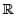and f(0) = 1, f'(0) = 0 and f"(0) = – 1. Then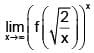is _______ (correct up to three decimal places).

*Answer can only contain numeric values
Math - 2018 Past Year Paper - Question 53

Suppose x, y, z are positive real number such that x + 2y + 3z = 1. If M is the maximum value of xyz2, then the value of 1/M is _______ .

*Answer can only contain numeric values
Math - 2018 Past Year Paper - Question 54

If the volume of the solid in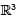bounded by the surfaces x = – 1, x = 1, y = – 1, y = 1, z = 2, y2 + z2 = 2 is α– π, then a = _______ .

*Answer can only contain numeric values
Math - 2018 Past Year Paper - Question 55

If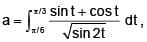then the value of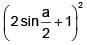is _______ .

*Answer can only contain numeric values
Math - 2018 Past Year Paper - Question 56

The value of the integral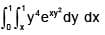is _______ . (correct up to three decimal places).

*Answer can only contain numeric values
Math - 2018 Past Year Paper - Question 57

Suppose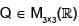is a matrix of rank 2. Let T :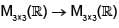be the linear transformation defined by T(P) = QP. Then the rank of T is _______ .

*Answer can only contain numeric values
Math - 2018 Past Year Paper - Question 58

The area of the parametrized surface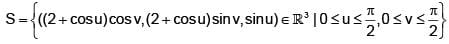is _______ (correct up to two decimal places).

*Answer can only contain numeric values
Math - 2018 Past Year Paper - Question 59

If x(t) is the solution to the differential equation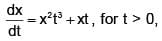satisfying x(0) = 1, then the value of x (√2 ) is _______ (correct up to two decimal places).

*Answer can only contain numeric values
Math - 2018 Past Year Paper - Question 60

If y(x) = v(x) sec x is the solution of y" – (2 tan x)y' + 5y = 0,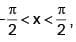satisfying y(0) = 0 and y '(0) =  √6, then v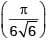is _______ . (correct up to two decimal places).

## IIT JAM Past Year Papers and Model Test Paper (All Branches)

48 tests
 Use Code STAYHOME200 and get INR 200 additional OFF Use Coupon Code
Information about Math - 2018 Past Year Paper Page
In this test you can find the Exam questions for Math - 2018 Past Year Paper solved & explained in the simplest way possible. Besides giving Questions and answers for Math - 2018 Past Year Paper, EduRev gives you an ample number of Online tests for practice

48 tests